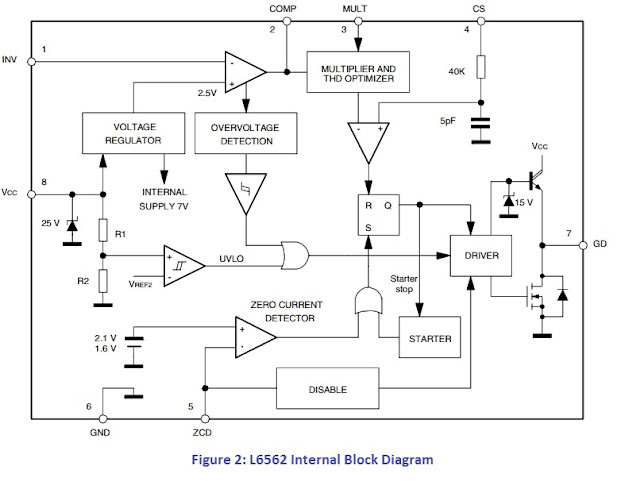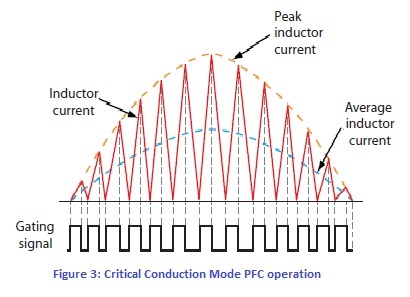# Power Factor Correction (PFC) – Working of PFC boost Converter using Critical Conduction Mode

### Power Factor Correction

Power Factor is a measurement of effectiveness of applied power, which is used by an electrical circuit or system. Power Factor Correction circuit or components improves power factor and increases efficiency of applied power supply.

More details on "Power Factor and Power Factor Correction", you can find on my previous blog and link for the same is;
https://www.powerelectronicstalks.com/2018/09/what-is-power-factor-correction.html

There are three modes for power factor correction; Continuous Mode, Discontinuous Mode and Critical Conduction Mode.

More details on "Modes of Operation of Power Factor Correction", you can find on my previous blog and link for the same is;
https://www.powerelectronicstalks.com/2018/10/power-factor-correction-and-its-modes.html

We know that Critical Conduction Mode based Power Factor Correction Circuit operates not exactly on, but very close to, the boundary between continuous and discontinuous current mode.

Below steps will clear the operation of the PFC boost converter in Critical Conduction Mode;
·        The Bridge rectifier rectifies the AC voltage and converts it into DC voltage and this DC voltage is fed to the boost converter.
·        Boost Converter uses a switching technique, which boosts the rectified input voltage to a regulated DC output voltage (DC bus).
·        The boost converter consists of a boost inductor (L1), a controlled power switch (M1), a flyback diode (D7), an output capacitor (C19) and a control circuitry (see Figure 1).PFC boost circuit using L6562

·        The purpose of this scheme is to shape the input current in a sinusoidal way, where it should be in phase with the input sinusoidal voltage.
·        To achieve this, L6562A uses the Critical Conduction Mode technique.
·        Please see Figure 2 for the L6562 Internal Block Diagram. We can see that, in L6562 the error amplifier compares a portion of the output voltage of the boost converter with an internal reference, and then generates an error signal which is proportional to the difference between them.
·        If the bandwidth of the error amplifier is sufficiently narrow (below 20 Hz), the error signal is a DC value over a given half-cycle.L6562 Internal Block Diagram

·        Further the error signal is fed into the multiplier block and multiplied by a portion of the rectified mains voltage.
·        The output of the multiplier is a rectified sinusoid whose peak amplitude depends on the mains peak voltage and the value of the error signal.
·        The output of the multiplier now fed into the (+) input of the current comparator; it represents a sinusoidal reference for PWM. In fact, as the voltage on the current sense pin (instantaneous inductor current times the sense resistor) equals the value on the (+) of the current comparator, the conduction of the MOSFET is terminated.
·        As a result, the peak inductor current is enveloped by a rectified sinusoid. Critical Conduction Mode control causes a constant ON-time operation over each line half-cycle.
·        When MOSFET turned off, the boost inductor discharges its power into the load until its current goes to zero.
·        The boost inductor has now run out of power, the drain node of MOSFET is floating and the inductor resonates with the total capacitance of the drain.
·        The drain voltage drops rapidly below the immediate line voltage and the signal on ZCD drives the MOSFET on again and another conversion cycle starts.
·        This low voltage across the MOSFET at turn-on reduces both the switching losses and the total drain capacitance energy that is dissipated inside the MOSFET.
·        The resulting inductor current and the Gating signals of the MOSFET are shown in Figure 3. We can also see that where it is also shown that, the average inductor current is just half of the peak inductor current waveform.Critical Conduction Mode PFC operation

### Conclusion

Critical Conduction Mode based Power Factor Correction circuit is widely used method for power factor correction. It works at boundary between continuous and discontinuous current mode. Implementing it with boost converter gives good power factor correction for many mid-wattage applications.

Reference : Referred STMicroelectronics "L6562 TRANSITION-MODE PFC CONTROLLER". Link is at below;
https://www.st.com/resource/en/datasheet/l6562.pdf